Guo, Y., Zhang, Y., Liu, M., Chen, X., and Tian, X. (2022). "Kinetics of delignification and carbohydrate degradation during the ozone bleaching of low-consistency hardwood pulps," BioResources 17(1), 429-444.

#### Abstract

With rising concern for environmental protection, the use of ozone has been increasingly studied in the pulp and paper industry. Feasible models for efficient quality prediction and process control are in high industrial demand. In this study, the reaction kinetics of delignification and viscosity during the ozone bleaching process (OBP) of low-consistency hardwood pulp (LCHP) are explored using exponential and zero-order response models, respectively. The effects of ozone dose, reaction temperature, reaction time, and pH on the changes in residual lignin content and pulp viscosity were analyzed. The corresponding kinetic parameters, such as the reaction order, rate constant (k), and activation energy (E), were also obtained. The models suggest that temperature should be one of the most significant factors affecting the effectiveness of the OBP system. The strategy to improve the OBP selectivity is based on reducing the reaction temperature while increasing the ozone concentration and pH in the reaction system.

Kinetics of Delignification and Carbohydrate Degradation during the Ozone Bleaching of Low-consistency Hardwood Pulps

Yixuan Guo,a,# Ye Zhang,b,# Mingyou Liu,a Xia Chen,a and Xiaofei Tian b,*

With rising concern for environmental protection, the use of ozone has been increasingly studied in the pulp and paper industry. Feasible models for efficient quality prediction and process control are in high industrial demand. In this study, the reaction kinetics of delignification and viscosity during the ozone bleaching process (OBP) of low-consistency hardwood pulp (LCHP) are explored using exponential and zero-order response models, respectively. The effects of ozone dose, reaction temperature, reaction time, and pH on the changes in residual lignin content and pulp viscosity were analyzed. The corresponding kinetic parameters, such as the reaction order, rate constant (k), and activation energy (E), were also obtained. The models suggest that temperature should be one of the most significant factors affecting the effectiveness of the OBP system. The strategy to improve the OBP selectivity is based on reducing the reaction temperature while increasing the ozone concentration and pH in the reaction system.

DOI: 10.15376/biores.17.429-444

Keywords: Hardwood pulp; Low-concentration pulp; Ozone bleaching; Optimization; Kinetic modeling

Contact information: a: School of Light Industry, South China University of Technology, 381 Wushan Road, Guangzhou, 510006, China; b: School of Biology and Biological Engineering, South China University of Technology, University Park, Guangzhou, 510006, China;

#: Co-first authors; *Corresponding author: xtien@scut.edu.cn

INTRODUCTION

In the pulp and paper industry, chlorine-based bleaching agents have been used for more than a century. With the increasingly strict requirements for environmental protection, the environmental hazards of the chlorinated organics from chlorine-based bleaching of wastewater have caused widespread concern. Currently, bleaching processes based on chlorine and hypochlorite have been restricted, and the industry has been moving toward elemental chlorine-free bleaching methods (Sharma et al. 2020). Ozone is a highly efficient, non-chlorine bleaching reagent. It has been gradually introduced into the pulp bleaching industry since the mid-1970s.

The ozone bleaching process (OBP) has the advantages of a low reaction temperature, short time, and high efficiency (Valcheva et al. 1999). The process is also highly adaptable for a variety of pulps. After bleaching, only degraded residuals of lignin and carbohydrate are found in the wastewater. In recent years, the industrialization of OBPs has become feasible with the advancements of ozone generators and OBP reactors (Chen et al. 2006), as well as the development and optimization of deep ozone delignification and pre-bleaching technology (Grossmann and Salmén 1997; Musl et al. 2019).

It was reported that the OBP was employed in more than 20 pulp mills worldwide. Ozone could be used in both Totally Chlorine Free (TCF) and Elemental Chlorine Free (ECF) bleaching sequences. With the advantages of high adaptability and efficiency but low operating temperature, ozone bleaching can effectively remove the hexenyl glycolic acid from the pulp to improve the stability of whiteness and reduce the dissolved polluting emission in the liquid effluent.

The most attractive implementation of ozone bleaching is in a TCF sequence for hardwood kraft pulp. However, the viscosity loss of TCF bleaching sequences, such as Z-E-P and Z-E-P-P, is usually attributed to free radicals of ozone produced by the reaction between ozone and lignin, which could depolymerize the cellulose. To apply ozone bleaching in both an efficient and a selective way is a common concern of the pulp industry (Ragnar et al. 2005; Pouyet et al. 2013). During the OBP, the reaction rate and bleaching efficiency can be affected by various factors. The OBP optimization has been extensively investigated. However, the relationship between key reaction factors and the delignification rate and the reduction of pulp viscosity have rarely been studied in OBPs. Bleaching kinetics studies have generally focused on processes with oxygen (Jablonský et al. 2013), chlorine dioxide (Lei et al. 2020), and hydrogen peroxide (Sun et al. 2018). Tran (2006) and Chapman et al. (1998, 1999) have reported the OBP kinetics of high-consistency pulp. He et al. (2018) reported that the mass transfer rate of ozone in water can greatly improve the performance of OBPs. However, the kinetics for OBP of low-consistency pulp (LCP) have not been established. Through a kinetic study, it is possible to understand the detailed regulation between the reaction of ozone and pulp. It is also important for efficient reaction control to improve the quality of pulp by promoting the delignification rate and reducing the degradation rate of carbohydrate polymers.

It is well known that ozone reacts rapidly with lignin because of its strong oxidizing properties. Consequently, the transfer resistance must be considered when studying the kinetics of OBP. Ozone bleaching is a complex mass transfer process, which includes both gas-liquid (undergoing autohydrolysis and oxidation reactions) and solid chemical-absorption mass transfers. In the bleaching process of low-concentration pulp, the presence of a large amount of aqueous solution makes the fibers more dispersed. The mass transfer during OBP can be simplified by a diffusion model for chemical absorption in dilute solutions based on two-film theory (Fig. S1) (McCabe et al. 1993).

According to the two-film theory, the ozone passes toward the pulp surface through gas (ozone) and liquid (water) films by molecular diffusion. When the resistance to mass transfer at the gas-liquid interface is assumed to be zero, the concentrations of ozone in the gas (CG, kmol/m³) and liquid (CL*, kmol/m³) films, which are in phase equilibrium, can be calculated using Eq. 1 (McCabe et al. 1993),

CG = m × CL* (1)

where m is the allocation coefficient.

In addition, the relationship between the total-mass-transfer and the sub-mass-transfer coefficients is shown by Eq. 2 (McCabe et al. 1993),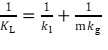(2)

where kL (m²/s) is the total mass transfer coefficient, kl (m²/s) is the liquid phase mass transfer coefficient, and kg [kmol/(m·s·kPa)] is the gas phase mass transfer coefficient.

In a low-concentration pulp, the fibers are well suspended in water. The ozone diffusion in the immobilized water layer on the fiber wall is rate-determined. According to the Osawa-Schuerch model (Fig. S2) (Roncero et al. 2003), ozone transfer generally occurs in the liquid phase before reaching the reaction site on the fiber.

Because ozone is relatively insoluble in water, the resistance in the gas phase can be considered negligible. The liquid film mainly contributes to the mass transfer resistance. The values of 1/kl should be larger than 1/m·kg. There is little difference between surface kL (m²/s) and kl (m²/s). The promotion of the transfer rate relies on reducing the liquid film resistance based on the liquid film control model (Fig. S3) (Griffin et al. 1998).

Therefore, the ozone dose transferred to the pulp can be calculated by Eq. 3,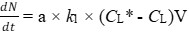(3)

where N [kmol/(m²·s)] is the mass transfer flux of the solute, a is the interfacial area, CL* (kmol/m³) is the concentration of solute in equilibrium at the liquid-phase interface, CL (kmol/m³) is the concentration of solute at the liquid-phase interface, and V (m/s) is the velocity.

With CL = N/V, Eq. 3 can be simplified to Eq. 4: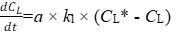(4)

During the transfer of ozone from a gas phase of known composition to an aqueous medium, the determination of kl×a in the nonstationary state can be accomplished by measuring the ozone concentration in the aqueous phase as a function of time (Griffin et al. 1998). In addition to reacting with lignin, ozone can also react with polysaccharides during the OBP. The kinetics of delignification and carbohydrate degradation should both be studied (Jablonský et al. 2004). The reaction rate can be affected by various experimental factors in the non-equilibrium dynamic system (Roncero et al. 2003).

In this work, time-course changes in the lignin composition and viscosity of pulp at various reaction temperatures, ozone doses, and pH values were investigated. Kinetic parameters, such as the reaction order, rate constant k, and activation energy, were obtained by the regression method. Kinetic models for delignification and carbohydrate degradation during the OBP of LCP are established. These kinetic models can provide a theoretical basis for the optimization and practical control of the OBP parameters to improve the quality of bleached pulp.

EXPERIMENTAL

Materials and Equipment

Kraft hardwood pulp was provided by Hongyuan Pulp and Paper Co., Ltd. (Fangchenggang, Guangxi, China). The Kappa number, viscosity, and brightness of the pulp were 2.5, 825 mL/g, and 30% ISO, respectively. The OBP system (Fig. S4) includes a KCF-SF100B ozone generator (Jiangsu Kanger Ozone Co., Ltd., Jiangsu, China), gas flowmeter (Jiangsu Kanger Ozone Co., Ltd., Jiangsu, China), ozone concentration detector (Jiangsu Kanger Ozone Co., Ltd., Jiangsu, China), ozone reactor (Dawn Precision Instrument Co., Ltd., Yantai, China), and residual gas absorption bottle with 20% (w/w) KI solution. A 13.5 ± 0.5 MPa oxygen (99.2%, Shengying Gas Co., Ltd., Guangzhou, China) was used as the gas source.

Methods

Ozone bleaching process

Briefly, 30 g of dry pulp was evenly dispersed and mixed with 15 mL of additive solution containing 5% (w/v) carbamic acid and 5% (w/v) N-hydroxy-ethyl ester. The pH of the mixture was adjusted to the designated value with 10% (w/w) sulfuric acid. The mixture was diluted to a pulp consistency of 3% (w/w) and loaded in the reactor at a stirring speed of 750 r/min. The amount of residual ozone collected by the KI solution was calculated by the titration method using Na2S2O3 (Torres et al. 2004).

Evaluating the reacted ozone

The actual amount of the reacted ozone was calculated by Eq. 5 below (Torres et al. 2004):

Mass of reacted ozone (g) = Mass of supplied ozone (g) – Mass of ozone collected (g) (5)

Pulp test and analyses

The OBP was conducted at ozone doses of 0.4% to 1.2% (w/w), reaction times of 5 min to 40 min, pH of 2.0, 2.5, and 3.0, as well as temperatures of 25 °C, 30 °C, and 35 °C. The determinations of moisture, Kappa number, viscosity, and brightness of the bleached pulp were made following TAPPI T412 om-02 (2002), TAPPI T236-om-99 (1999), TAPPI T230 om-99 (1999), and TAPPI T217 wd-77 (2004) standards, respectively. Data analysis was obtained by the software Origin (OriginLab Corporation, Origin 9.1, Northampton, MA, USA).

Kinetic Modeling for the Delignification of LCP in the OBP

The delignification exponential model (Eq. 6) (Ji et al. 2007) was applied in this study,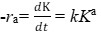(6)

where ra is the reaction rate, k is the reaction rate constant, K is the pulp Kappa number, and ‘a’ is the delignification reaction order.

It is known that ozone delignification is strongly influenced by the reaction temperature, ozone dose, and pH. Therefore, the reaction rate ra is a function of temperature, ozone dose, and pH. Because pH = -log[H+], the H+ concentration is dependent on the initial pH value. Thus, Eq. 6 can be converted to Eq. 7 (Roncero et al. 2012),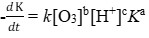(7)

where b is the reaction order of ozone consumption and c is the reaction order of H+ concentration.

According to the collision theory of the chemical reaction rate, any chemical reaction has a minimum critical energy, and the reaction can only occur when the energy of the reactant molecule exceeds this minimum energy. Similar to other chemical reactions, the OBP is also affected by the reaction temperature, which reflects the average energy of molecules. According to the Arrhenius equation (Iribarne and Schroeder 1997), the relationship between the reaction rate constant k and temperature (T, °C) is: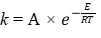(8)

When Eq. 8 is substituted into Eq. 7, Eq. 9 can be acquired,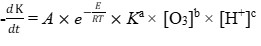(9)

where K is the Kappa number of the pulp, t is the ozone bleaching reaction time (min), A is the pre-exponential factor, E is the reaction activation energy (kJ/mol), R is the gas constant, 8.314 J·mol-1K-1, and [O3] is the ozone dose (%). Because pH = -log[H+], the H+ concentration is dependent on the initial pH value; furthermore, ‘a’ is the delignification reaction order, ‘b’ is the reaction order of ozone consumption, and ‘c’ is the reaction order of H+ concentration.

Thus, Eq. 6 can be integrated to produce Eq. 10: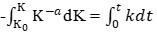(10)

Equation 10 can be solved to obtain Eq. 11,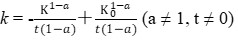(11)

where k is the reaction rate constant, K0 is the initial Kappa number, and ‘a’ is the order of the delignification reaction.

According to the changes in the Kappa number over time at various experimental temperatures, the reaction rate constant k and the delignification reaction order ‘a’ at different temperatures are obtained by fitting Eq. 11.

Equation 12 can be obtained through the logarithmic transformation of Eq. 9: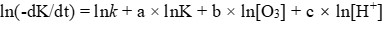(12)

The relationship between temperature, ozone dose, and reaction rate can be determined by fixing the levels of the other two factors. The respective reaction order can also be acquired.

Kinetic Modeling for Carbohydrate Degradation during OBP

During OBP, the ozone not only can react with lignin but also it can destroy the carbohydrate polymeric structure, resulting in reduced pulp viscosity and paper strength (Johansson et al. 2000). To minimize the degradation of carbohydrates in the OBP, additives can be used to protect the pulp viscosity (Roncero et al. 2003). Studying the reaction rate of carbohydrate polymer degradation would help to resolve the degradation of the carbohydrate polymer from the perspective of reaction kinetics.

The content of carbohydrate polymers is usually recognized by Mn, which represents the moles of cellulose chains per metric ton of pulp. This value can be quantitatively calculated by Eq. 13 (Doǧan and Gürüz 2008),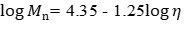(13)

where η is the experimentally measured viscosity of the pulp.

According to the limited kinetic models with respect to a zero-order reaction for carbohydrate degradation (Doǧan and Gürüz 2008), the zero-order kinetic model (Eq. 14) was adopted in this study,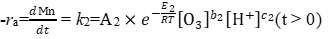(14)

where k2 is the reaction rate constant, Mn is the moles of cellulose chains per metric ton of pulp (mol/t), A2 is the pre-exponential factor, T is the temperature (°C), b2 is the reaction order of ozone consumption, and c2 is the reaction order of H+ concentration.

When the ozone dose and H+ concentration are constant, Eq. 14 can be converted into Eq. 15,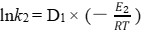(15)

where k2 is the reaction rate constant and D1 is a constant, which means that the product of the ozone dose and H+ concentration is constant regardless of the temperature change. The activation energy E2 for carbohydrate degradation can be obtained from the slope of the linear regression between lnk2 and 1/T.

Thus, Eq. 14 can also be integrated to obtain Eq. 16: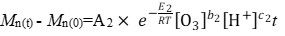(16)

For a zero-order reaction, Mn and t are linearly correlated with the reaction rate constant k3 when both the temperature and H+ concentration are constant. The resulting expression (Eq. 17) is as follows,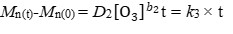(17)

where D2 is a constant, which means that the product of temperature and H+ concentration is constant regardless of the variation in ozone dose.

Similarly, when the temperature and ozone dose are constant, Eq. 16 can be converted to Eq. 18,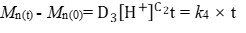(18)

where D3 is a constant, which means that the product of temperature and ozone dose is constant regardless of the change in H+ concentration, and k4 is the reaction rate constant.

Validation Test of the Kinetic Models

The goodness of fit was assessed through a visual inspection of the model predictions in comparison with the experimental data. Additionally, the strength of the relationship between the trend of the predicted values and the trend of the experimental values was determined by calculating the correlation coefficient (r) of the predicted and experimental values c by using SPSS Statistics 20 (IBM corporation, NY, USA).

RESULTS AND DISCUSSION

Delignification Kinetics during the OBP

The lignin content of pulp is usually indirectly indicated by the Kappa number of the pulp. In the OBP, the parameters that affect the chemical reaction rate were pulp consistency, pH, ozone dose, stirring rate, stirring time, additives, and reaction temperature. Therefore, the removal of residual lignin in the OBP of LCP by changing the pH, ozone dose, and reaction temperature was investigated in this study.

Determination of the delignification reaction order

Because of its strong oxidation capacity, ozone was not stable and could be easily decomposed by the influence of a high temperature. Therefore, a lower temperature favored the OBP efficiency (Allison 1985; Tripathi et al. 2020). The Kappa number also decreased rapidly with decreasing reaction temperature. Moreover, a proper reaction time was beneficial to the ozone bleaching efficiency by reducing the ineffective decomposition of ozone (Dong 2017). As the reaction time progressed, within the range 25 to 35 °C, the Kappa number gradually decreased from the initial value of 12.5. As the declining utilization rate of ozone for delignification, the decrease of the pulp Kappa number dramatically slowed down after a 30-min reaction time. (Fig 1a). The -dK/dt value could be obtained by derivation of the linear regression formula using the K and t.

When the ozone dose and H+ concentration are at a certain level, C1 is a constant:

C1 = lnk + b × ln[O3] + c × ln[H+] (19)

From Eqs. 12 and 19, Eq. 20 can be obtained:

ln(-dK/dt) = C1 + a × lnK (20)

From the linear regression of Eq. 20, the slope denotes the reaction order of ozone delignification (Fig. 1b). The calculated average reaction order was a = 6.469.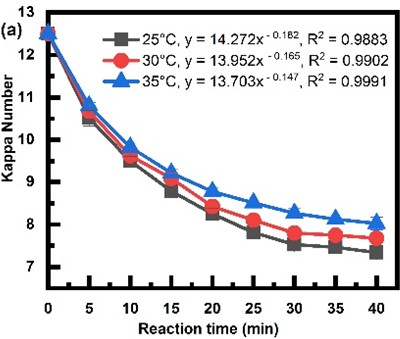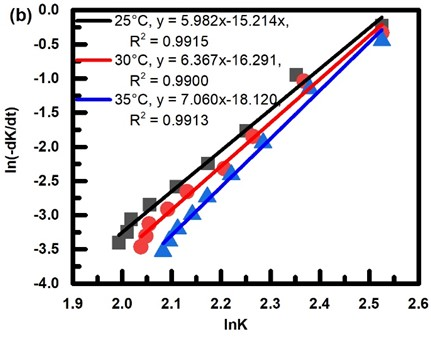Fig. 1. Change in the Kappa number over time at different temperatures: a) Reaction conditions: ozone dose of 1.0%, pulp consistency of 3% (w/w), and pH = 2.0; b) Linear regression plotting of ln(-dK/dt) and lnK

Effect of the ozone dose and H+ concentration on the delignification kinetics

Figure 2 shows that as the reaction time increases, the Kappa number of the pulp gradually decreases from the initial value of 12.5. At a certain reaction time, the decrease in the Kappa number of the pulp is accompanied by an increase in the ozone dose (Fig. 2a) or a decrease in hydrogen ion concentration (Fig. 2b). The corresponding regressions between the Kappa number and time can be found.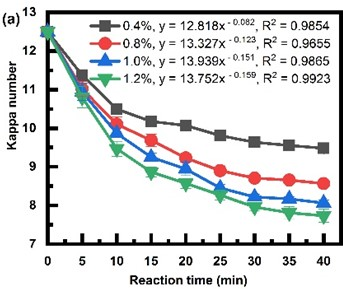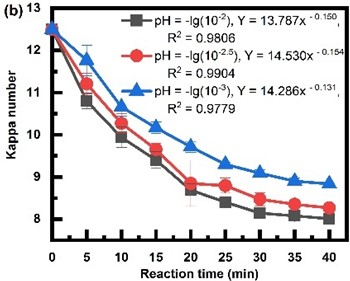Fig. 2. Changes in the Kappa numbers against the reaction time at different ozone doses and pH;

reaction conditions: pulp consistency of 3% (w/w), temperature 35 °C, a 0.5% (w/w) additive solution, and a) pH = 2.0, b) ozone dose 1.0%

When the H+ concentration and reaction temperature are at a certain level, a constant C2 can be defined as:

C2 = lnk + c × ln[H+] (21)

From Eqs. 12 and 21, Eq. 22 can be obtained:

ln(-dK/dt) – a × lnK = C2 + b × ln[O3] (22)

Similarly, when the ozone dose and reaction temperature are at a certain level, a constant C3 can be defined as:

C3 = lnk + b × ln[O3] (23)

From Eqs. 12 and 23, Eq. 24 can be obtained:

ln(-dK/dt) – a × lnK = C3 + c × ln[H+] (24)

By linear regression (Eqs. 22 and 24), the slopes b and c are obtained, which are the delignification reaction orders relative to the ozone dose (Fig. 3a) and H+ concentration (Fig. 3b), respectively. The calculated average reaction orders are b = 1.3755 and c = 0.283.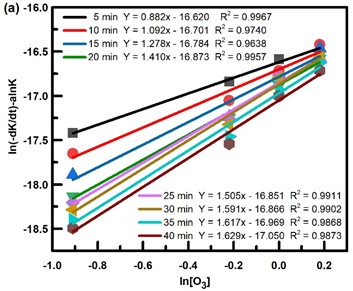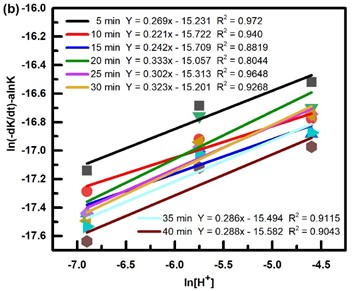Fig. 3. Linear regression plotting between [ln(-dK/dt)-alnK] and (a) ln[O3] or (b) ln[H+]

Effect of temperature on the delignification kinetics

According to Eq. 12, when the Kappa value, ozone dose, and H+ concentration are constant, Eq. 25 can be obtained,

lnk = ln(-dK/dt) – a × lnKb × ln[O3] – c × ln[H]+ (25)

where a = 6.469, b = 1.3755, and c = 0.283.

The lnk values at the three temperatures used are calculated (Table 1)

Table 1. Calculated lnk at Three Different Temperatures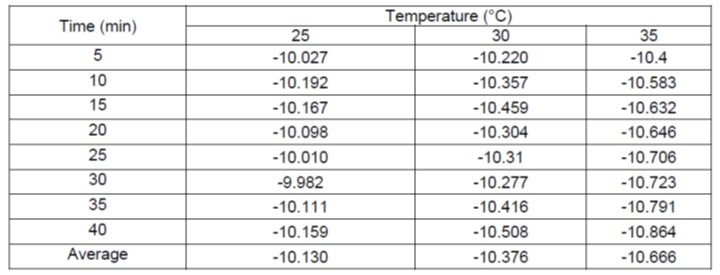Equation 26 can be obtained from the derivation of Eq. 8:

lnk = lnAE/RT (26)

Equation 26 revealed that there existed a linear relationship between the lnk and the reciprocal of time (1/T). The equation lnk = 4.906(1/T) – 26.5761 (R² = 0.9959) could be acquired by the regression of the average lnk values and corresponding 1/T in Table 1. Therefore, E/R = -4.906 × 103, and E = 40.788 kJ/mol.

Kinetic model for delignification during the OBP

Through substituting the obtained a, b, and c values into Eq. 5, the kinetic equation for the OBP of LCHP can be obtained as Eq. 27,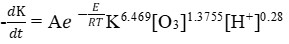(27)

where the reaction activation energy is calculated as E = 40.788 kJ/mol.

In the process of OBP, ozone not only reacts with lignin but it also degrades carbohydrates, resulting in reduced pulp viscosity and paper strength. To minimize the degradation of carbohydrates during the bleaching process, the reaction rate of carbohydrate degradation should be studied during the ozone bleaching reaction from the point of view of reaction kinetics. In this study, the degradation of carbohydrates is indirectly represented by the length of cellulose chains, and the degradation of carbohydrates during the OBP of LCHP is investigated at various pH values, reaction temperatures, and ozone doses.

Effect of temperature, ozone dose, and H+ concentration on the kinetics of carbohydrate degradation

Figures 4a, b, and c show that the cellulose molecular weight (Mn) gradually increased with an increasing reaction time. At certain reaction times, increasing temperature, ozone dose, and pH led to increases in the cellulose chains. The high temperature, ozone dose, and pH accelerated the decomposition of ozone into hydroxide and peroxyl free radicals, which could efficiently reduce the viscosity of the pulp by breaking down carbohydrate polymers; thus, the cellulose chain increased.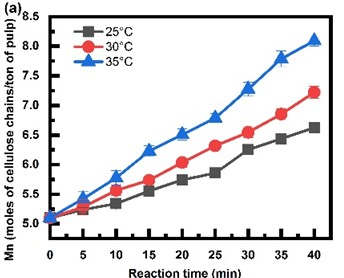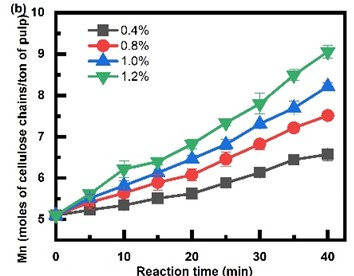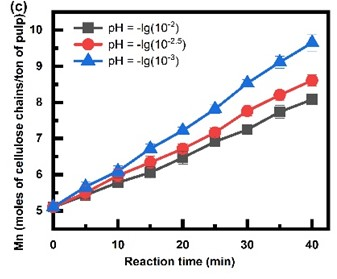Fig. 4. Changes in the cellulose molecular weight against reaction time; reaction conditions: a) pulp consistency of 3% (w/w), ozone dose of 1.0% (w/w), pH = 2.0, and a 0.5% (w/w) additive solution; b) pulp consistency of 3%, pH = 2.0, temperature of 35 °C, and a 0.5% additive solution; c) pulp consistency of 3%, ozone dose of 1.0%, temperature of 35 °C, and a 0.5% additive solution

Figures 4a, b, and c show that the degradation rate of carbohydrate polymers could be different between the initial and late stages throughout the OBP. Similar to the oxygen delignification process, the degradation kinetics of carbohydrates could also be divided into multiple stages (Iribarne and Schroeder 1997). Through linear regression of the cellulose chain Mn and reaction time, the relatively high R2 obtained also showed that the zero-order reaction model was applicable to the degradation of carbohydrates during the OBP (Tables 2 and 3). The degradation rate constants k2, k3, and k4 could be acquired from the slopes of the equations.

Table 2. Linear Regression of the Cellulose Chains Over Time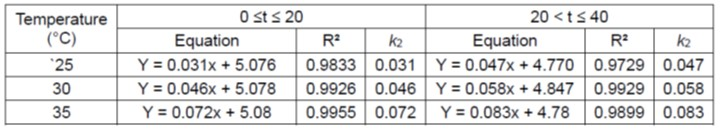Table 3. Linear Regression of the Cellulose Chains Over Time at Different Ozone Doses and pH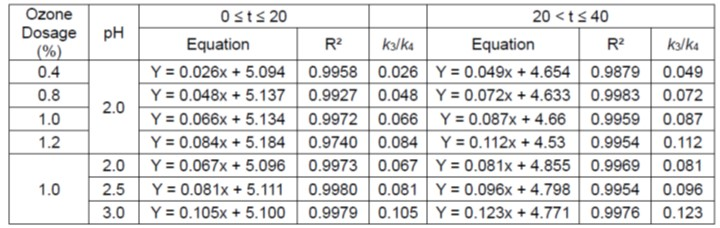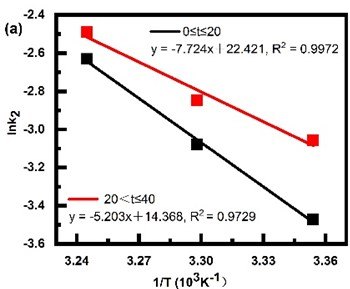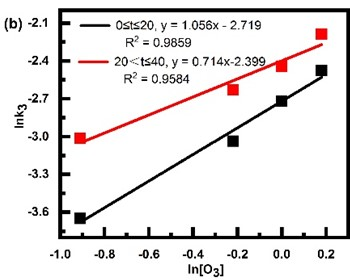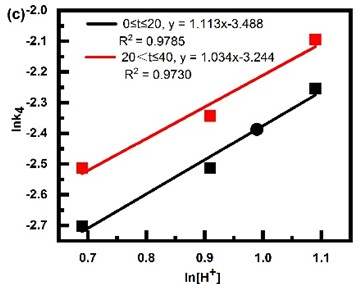Fig. 5. Plots of a) lnk2 versus 1/T; b) lnk3 versus ln[O3]; and c) lnk4 versus ln[H+]

According to Eq. 15, the regression between lnk2 and -1/T is shown in Fig. 5a. Two linear regression equations, i.e., y = -7.724x + 22.421 (0 ≤ t ≤ 20) and y = -5.203x +14.368 (20 < t ≤ 40), were obtained. Through the slope, the calculated E2 and E3 values were 64.217 kJ/mol (0 ≤ t ≤ 20) and 43.257 kJ/mol (20 < t ≤ 40), respectively.

The carbohydrate degradation reaction order b2 and c2 were affected by the ozone dose, and the H+ concentration could be obtained by taking the logarithms of Eqs. 17 and 18. Through linear regression, the correlations between lnk3 and -1/T and lnk4 and -1/T are shown in Fig. 5b and c, respectively.

The order b2 could be acquired from the slope of y = 1.056x – 2.719 (0 ≤ t ≤ 20) and y = 0.714x – 2.399 (20 < t ≤ 40), while c2 could be acquired from the slope of y = 1.113x – 3.488 (0 ≤ t ≤ 20) and y = 1.034x – 3.244 (20 < t ≤ 40).

Substituting the above obtained values of E2, E3, b2, and c2 into Eq. 9, the kinetic equation of carbohydrate degradation during the OBP of LCHP can be established as Eq. 28,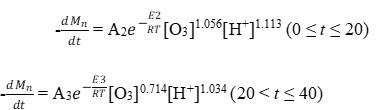(28)

where E2 = 64.217 kJ/mol, E3 = 43.257 kJ/mol, and t is the reaction time (min).

Validation of the Model

As shown in Fig. 6, the trend of experimental value was found to be closely in agreement with the trend of predicted value from the models with high correlation coefficients of 0.996, 0.99, and 0.976, validating the effectiveness of the kinetic models of the delignification and carbohydrate degradation during OBP of LCHP. The model can well predict the pulp kappa number and viscosity under different conditions in the OBP, which provides theoretical guidance for the setting and control of OBP conditions for LCHP.

Process Optimization by Using the Established Models

Techno-economic analysis (TEA) was one of the most recognized tools for evaluating the feasibility and viability of the OBP, as well as its process optimization. TEA requires integrating all the key-dependent and independent factors relating to technical and economic performance.

By revealing the relationships between the change of pulp quality and other independent factors, such as operation time, energy input, and chemical consumption, the establishment of the kinetic models should make the process simulation and economic modeling possible for the TEA in the future.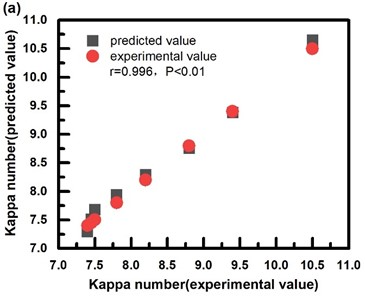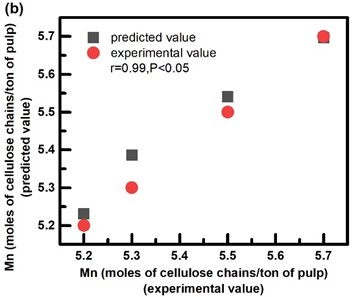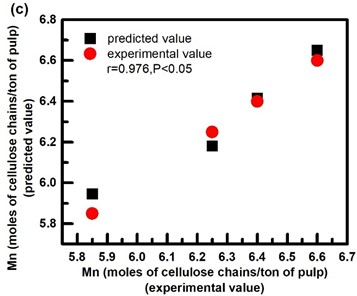Fig. 6. Correlations of the trend of experimental values and the predicted values of the delignification model; b) the carbohydrate degradation model (when 0 ≤ t ≤ 20) ; c) , and the carbohydrate degradation model (when 20 < t ≤ 40)

CONCLUSIONS

1. Kinetic models for delignification and carbohydrate degradation in the ozone bleaching process (OBP) of low-consistency hardwood pulp (LCHP) were established using controllable parameters of pH, ozone dosage, reaction time, and temperature. The effectiveness of the kinetic models was also validated in accordance with the experimental data. The high reaction order of the Kappa number (a=6.469) indicates that ozone has an advanced mass transfer efficiency in the bleaching process.
2. The non-integer kinetic orders of the Kappa number, ozone dose, and pH against delignification, as well as the reaction-time-dependent kinetic orders for carbohydrate degradation, indicated a complicated heterogeneous reaction process during the OBP of LCHP.
3. The models suggest that temperature should be one of the most significant factors affecting the effectiveness of the OBP system. The reaction temperature should be restricted, ideally from 25 to 30°C, and the ozone concentration (0.5% to 1.0%, w/w) should be properly controlled to ensure its sufficient contact to the lignin color-emitting groups, but also to avoid the self-decomposition of the bleaching agent during the gas distribution and mass transfers in the solution. A pre-soaking stage was also suggested by incubating the pulp with a pH level of 2 to stabilize the ozone molecules in the solution by decreasing its self-decomposition.
4. The strategy to improve OBP selectivity is based on reducing the reaction temperature while increasing the ozone concentration and pH in the reaction system.

ACKNOWLEDGMENTS

The authors are grateful for the financial support of Natural Science Foundation of Guangdong Province, China (Grant No. 2017A030316078); and Fundamental Research Funds for the Central Universities (Grant No. D2190870).

REFERENCES CITED

Chapman, A. A., Nguyen, K. L., and Cook, R. A. (1998). “Ozone bleaching of various eucalypt kraft pulps at high consistency,” in: 52nd APPITA Annual General Conference, Brisbane, Australia, pp. 353-360.

Chapman, A. A., Nguyen, K. L., and Cook, R. A. (1999). “A model for the kinetics of ozone bleaching at high consistency,” Journal of Pulp and Paper Science 25(7), 263-267.

Chen, H. L., Lee, H. M., and Chang, M. B. (2006). “Enhancement of energy yield for ozone production via packed-bed reactors,” Ozone: Science & Engineering 28(2), 111-118. DOI: 10.1080/01919510600559393

Doǧan, I., and Güniz, G. (2008). “Dimensionless parameter approach for oxygen delignification kinetics,” Industrial and Engineering Chemistry Research 47(16), 5871-5878. DOI: 10.1021/ie071498h

Dong, H. (2017). “Study on ozone bleaching of the ammonium sulfite wheat straw pulp,” Key Engineering Materials 753, 249-253. DOI: 10.4028/www.scientific.net/KEM.753.249

Griffin, R., Ni, Y., and Van Heiningen, A. (1998). “The development of delignification and lignin-cellulose selectivity during ozone bleaching,” Journal of Pulp and Paper Science 24(4), 111-115.

Grossmann, H., and Salmén, L. (1997). “Exploration of new scientific ideas for significant electricity savings in mechanical upgrading of pulp fibres for papermaking,” Applied Thermal Engineering 17(8–10), 947-954. DOI: 10.1016/s1359-4311(96)00056-7

He, T., Liu, M. Y., and Tian, X. (2018). “Kinetics of ozone bleaching of eucalyptus kraft pulp and factors affecting the properties of the bleached pulp,” BioResources 13(1), 425-436. DOI: 10.15376/biores.13.1.425-436

Iribarne, J., and Schroeder, L. R. (1997). “High-pressure oxygen delignification of kraft pulps – Part I: Kinetics,” TAPPI Journal 80(10), 241-250.

Jablonský, M., Lucia, K., Martin, A., and Botková, A. (2013). “The change of kinetics rate of excitation purity in ozonization of oxygen delignified hardwood kraft pulp,” Wood Research 58(3), 405-412.

Jablonský, M., Vrška, M., and Katuščák, S. (2004). “Cellulose protectors for improving ozone bleaching – Review,” Wood Research 49(4), 71-85.

Ji, Y., and Van Heiningen, A. (2007). “A new CSTR for oxygen delignification mechanism and kinetics study,” Pulp & Paper-Canada 108(5), 38-42.

Johansson, E., Lind, J., and Ljunggren, S. (2000). “Aspects of the chemistry of cellulose degradation and the effect of ethylene glycol during ozone delignification of kraft pulps,” Journal of Pulp and Paper Science 26(7), 239-244.

Lei, M., Luo, B., Zhang, Q., Guo, C., Chi, M., Yun, X., Chen, C., Min, D., and Wang, S. (2020). “Kinetics of the reaction between a lignin model compound and chlorine dioxide,” Chemical Engineering Journal 393, article ID 124783. DOI: 10.1016/j.cej.2020.124783

McCabe, W. L., Harriott, P., and Smith, J. C. (1993). Unit Operation in Chemical Engineering (5th Ed.), McGraw-Hill, New York, NY, USA.

Musl, O., Holzlechner, M., Winklehner, S., Gübitz, G., Potthast, A., Rosenau, T., and Böhmdorfer, S. (2019). “Changing the molecular structure of kraft lignins – Ozone treatment at alkaline conditions,” ACS Sustainable Chemistry and Engineering 7(18), 15163-15172. DOI: 10.1021/acssuschemeng.9b01046

Pouyet, F., Lachenal, D., Das, S., and Chirat, C. (2013). “Minimizing viscosity loss during totally chlorine-free bleaching of hardwood kraft pulp,” BioResources 8(1), 238-249.

Ragnar, M, Dahllöf, H., and Lundgren, S. (2005). “Towards environmentally sustainable bleaching of kraft pulp. Evaluating the possible role of ozone,” APPITA Journal 58. 475-480.

Roncero, M. B., Torres, A. L., Colom, J. F., and Vidal, T. (2012). “TCF bleaching of wheat straw pulp using ozone and xylanase: Part B: Kinetic studies,” Bioresource Technology 87(3), 315-323. DOI: 10.1016/S0960-8524(02)00225-0

Roncero, M. B., Queral, M. A., Colom, J. F., and Vidal, T. (2003). “Why acid pH increases the selectivity of the ozone bleaching processes,” Ozone: Science & Engineering 25(6), 523-534. DOI: 10.1080/01919510390481838

Roncero, M. B., Colom, J. F., and Vidal, T. (2003). “Increasing the selectivity and efficiency of ozone bleaching in a TCF sequence – Part C,” APPITA Journal 56(3), 194-199.

Sharma, N., Tripathi, S. K., and Bhardwaj, N. K. (2020). “Utilization of sarkanda for making pulp and paper using elemental chlorine free and total chlorine free bleaching processes,” Industrial Crops and Products 149, article ID 112316. DOI: 10.1016/j.indcrop.2020.112316

Sun, X., Hou, Q., Shi, H., and Zhou, L. (2018). “Kinetic study on Iso-concentration hydrogen peroxide bleaching of poplar chemi-thermomechanical pulp by the process of continuous chemical supplement,” BioResources 13(3), 5408-5415. DOI: 10.15376/biores.13.3.5408-5415

TAPPI T217 wd-77 (2004). “Brightness of pulp,” TAPPI Press, Atlanta, GA, USA.

TAPPI T230 om-99 (1999). “Viscosity of pulp,” TAPPI Press, Atlanta, GA, USA.

TAPPI T236 om-99 (1999). “Kappa number of pulp,” TAPPI Press, Atlanta, GA, USA.

TAPPI T412 om-02 (2002). “Moisture in pulp, paper and paperboard,” TAPPI Press, Atlanta, GA, USA.

Torres, A. L., Roncero, M. B., Colom, J. F., Martínez, J. A., and Vidal, T. (2004). “Application of an experimental design to modeling of ozone bleaching stage in TCF processes,” Ozone: Science and Engineering 26(5), 443-451. DOI: 10.1080/01919510490507685

Tran, A. V. (2006). “High-consistency ozonation of hardwood kraft pulp,” Holzforschung 60(6), 685-690. DOI: 10.1515/HF.2006.115

Tripathi, S. K., Bhardwaj, N. K., and Ghatak, H. R. (2020). “Developments in ozone-based bleaching of pulps,” Ozone: Science & Engineering 42(2), 194-210. DOI: 10.1080/01919512.2019.1647407

Valchev, I., Valcheva, E., and Christova, E. (1999). “Kinetics of oxygen delignification of hardwood kraft pulp,” Cellulose Chemistry and Technology 33(3-4), 303-310.

Article submitted: August 2, 2021; Peer review completed: October 17, 2021; Revised version received and accepted: November 13, 2021; Published: November 23, 2021.

DOI: 10.15376/biores.17.1.429-444